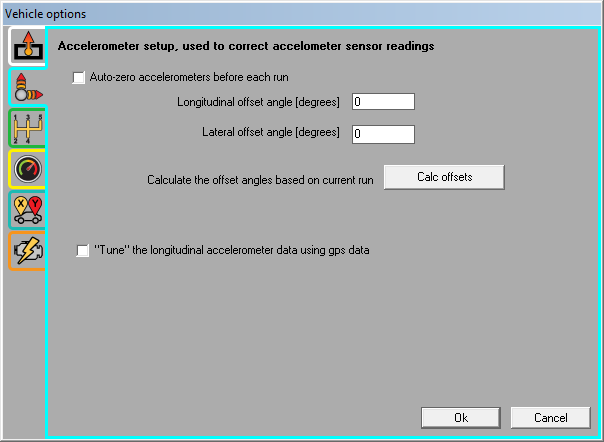Call me back |My basket | Checkout | Add to email listYou are here: Website » Knowledge base

# Accelerometers

Accelerometers are designed to measure acceleration in a particular direction. So to measure accelerations accurately it is essential that the logger or meter is accurately aligned in the car. In practice, this means that the unit must be mounted squarely in the vehicle and as level as possible. More details about mounting can be found here.

To allow for situations where the logger/meter cannot be mounted absolutely flat, there are a couple of options in the software that allow the accelerometer readings to be "adjusted" for the offset from horizontal. This is important since, if the accelerometers are not correctly adjusted for angle, then the accelerations will be wrong. For example, if the accelerometer is mounted at 10 degrees from the horizontal, then the acceleration reading will be offset by:

1g x sin(10 degrees) = 0.17g

and sensitivity will be reduced by:

1 - cos(10 degrees) = 2%

Clearly, such a large error on acceleration must be corrected. If in the case above nothing was done to correct the readings, then the acceleration reading would be almost meaningless. Further, the incorrect acceleration readings would also have knock on effects on the other acceleration-derived measurements, such as speed and power estimates and track mapping.

The zeroing options are available in the Vehicle Options. To access them, go to Data > Vehicle Options, and click on the Accelerometers tab. This will show the following:There are two methods that allow the accelerations to be corrected:

• "auto zero" the accelerometers at the beginning of the run.
• manually fix the mounting angles and "hardcode" this into the analysis.

In general, if the logger/meter is moved from the vehicle on a regular basis, then it is probably best to use the "auto zero" option. If the unit is to be permanently mounted in a vehicle, then entering fix angles into the analysis software will probably be more convenient.

If auto-zeroing is enabled, then the analysis software assumes that, for the first second of each run, the vehicle was standing stationary on a level surface and uses this information as a basis for calculating the mounting angle. This information is then used to correct the accelerations for the entire run. If there are multiple runs loaded into the software then this offset will be calculated for each individual run and applied only for that run.

Be particularly careful with the auto-zero option if you trim a run, if the start of the resultant trimmed run contains data with the vehicle moving or not level, then the accelerations will be incorrect.

If auto zeroing is enabled in the analysis and the vehicle is moving or the vehicle not level for the first second of the data then the accelerations (and derived variables) for the whole run will be incorrect.

The second method of entering angles manually obviously requires that you know the angle that the unit is mounted at. The simplest way of doing this is to park the car stationary on a level surface (or as near as possible) and log a few seconds of data. Disable the "auto zero" option in the analysis software and load in the data. With the data loaded and processed click the auto calculate option to automatically calculate the mounting angles. Once this has been done these mounting angles can be used for subsequent run analysis and the angles will remain valid unless the unit is moved. Note that this method calculates the angles based only on the first second of the first run that is loaded - all other data that is loaded has no effect on the result.

Finally is it worth mentioning about the maximum angle that you should mount the logger/accelerometer at; it's a common question that we get asked, and not one there is a simple answer to. Mathematically it is possible to correct the accelerations for any mounting angle except vertical, but in practice errors in the accelerations reading increase quite rapidly with mounting angle - as a rule of thumb it is best to limit the mounting angle to less than 10 degrees if possible. The problem with large mounting angles is 2 fold. Firstly in the equation above we assumed an offset of 1g x sin(10)=0.17g, but in practice the vertical acceleration is not a constant 1g as the vehicle has some vertical movement due to bumps and vibration. The errors due to the extra vertical accelerations cannot be removed from the data and look like noise on the data - filtering helps, but it does not eliminate the problem. The other problem is that with increasing mounting angles the sensitivity is reduced, so to compensate the acceleration measurements have to be multiplied up increasing noise and decreasing resolution.

Option to "tune" the longitudinal accelerometer offset using GPS data

This option uses the GPS information to "fine tune" the last remaining error from the accelerometers to ensure that you have the highest possible accuracy. If this option is enabled then the longitudinal accelerations are calculated from both the GPS speed information and the accelerometers, these 2 acceleration levels are compared and based on the result the accelerometers reading are corrected for small offsets. Please note that this option is not intended as a replacement for manually zeroing the accelerometers as described above - it should be used in addition and is only suitable for removing small residual errors. It is recommended that this option is used when accurate power figures are required.